• ## matplotlib隐藏坐标轴

千次阅读 2019-10-18 15:33:54
matplotlib隐藏坐标轴 matplotlib隐藏坐标轴: 1、方法一： # 隐藏坐标轴 pyplot.axes().get_xaxis().set_visible(False) pyplot.axes().get_yaxis().set_visible(False) 使用该方法会抛出警告，不影响使用： ...
matplotlib隐藏坐标轴
matplotlib隐藏坐标轴:
1、方法一：
    # 隐藏坐标轴
pyplot.axes().get_xaxis().set_visible(False)
pyplot.axes().get_yaxis().set_visible(False)

使用该方法会抛出警告，不影响使用：
 MatplotlibDeprecationWarning: Adding an axes using the same arguments as a previous axes currently reuses the earlier instance.
In a future version, a new instance will always be created and returned.
Meanwhile, this warning can be suppressed, and the future behavior ensured, by passing a unique label to each axes instance.
pyplot.axes().get_yaxis().set_visible(False)

2、方法二：
    # 隐藏坐标轴
pyplot.gca().get_xaxis().set_visible(False)
pyplot.gca().get_yaxis().set_visible(False)

展开全文python
• 学习https://matplotlib.org/gallery/index.html 记录，描述不一定准确，具体请参考官网 Matplotlib使用总结图 import matplotlib.pyplot as plt ...新建隐藏坐标轴 from mpl_toolkits.axisartist.
• #隐藏坐标轴 plt.axes().get_xaxis().set_visible(False)#不知道为什么隐藏坐标轴和突出起点和终点的代码没有用，有待观察 plt.axes().get_yaxis().set_visible(False) 仍然存在坐标轴 最后找到了解决办法： ...
    #隐藏坐标轴

plt.axes().get_xaxis().set_visible(False)#不知道为什么隐藏坐标轴和突出起点和终点的代码没有用，有待观察
plt.axes().get_yaxis().set_visible(False)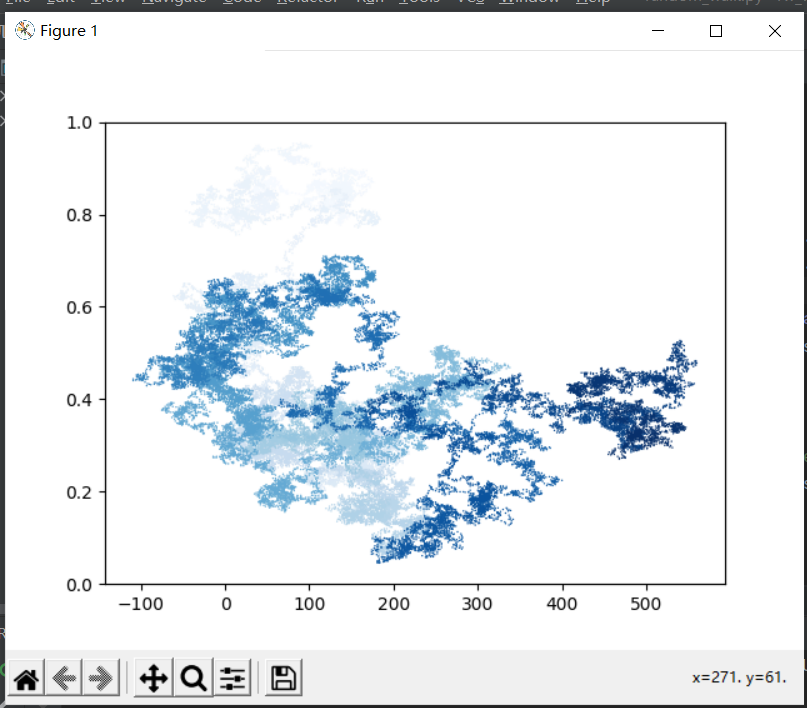仍然存在坐标轴
最后找到了解决办法：
必须将他赋值给一个变量，然后调用他。
current_axes = plt.axes()


    current_axes = plt.axes()
current_axes.get_xaxis().set_visible(False)
current_axes.get_yaxis().set_visible(False)

问题解决
同时，必须将隐藏坐标轴的代码置于scatter前。
展开全文python
• ## Matplotlib去掉坐标轴

万次阅读 多人点赞 2019-05-28 21:54:08
plt.xticks([]) #去掉x轴 plt.yticks([]) #去掉y轴 plt.axis('off') #去掉坐标轴
plt.xticks([])  #去掉x轴
plt.yticks([])  #去掉y轴
plt.axis('off')  #去掉坐标轴

或
ax = plt.gca()
ax.spines['top'].set_visible(False)
ax.spines['bottom'].set_visible(False)
ax.spines['left'].set_visible(False)
ax.spines['right'].set_visible(False)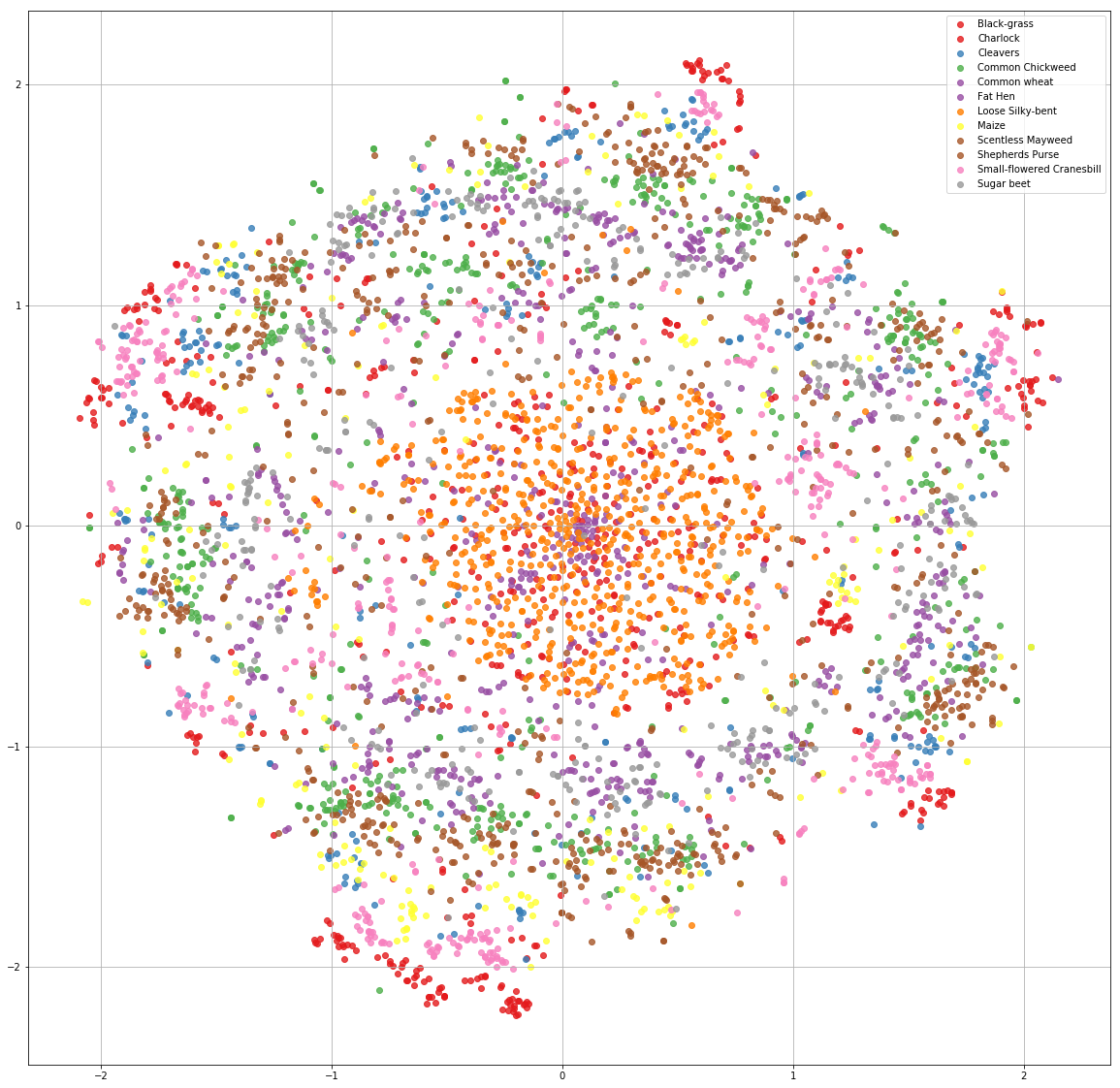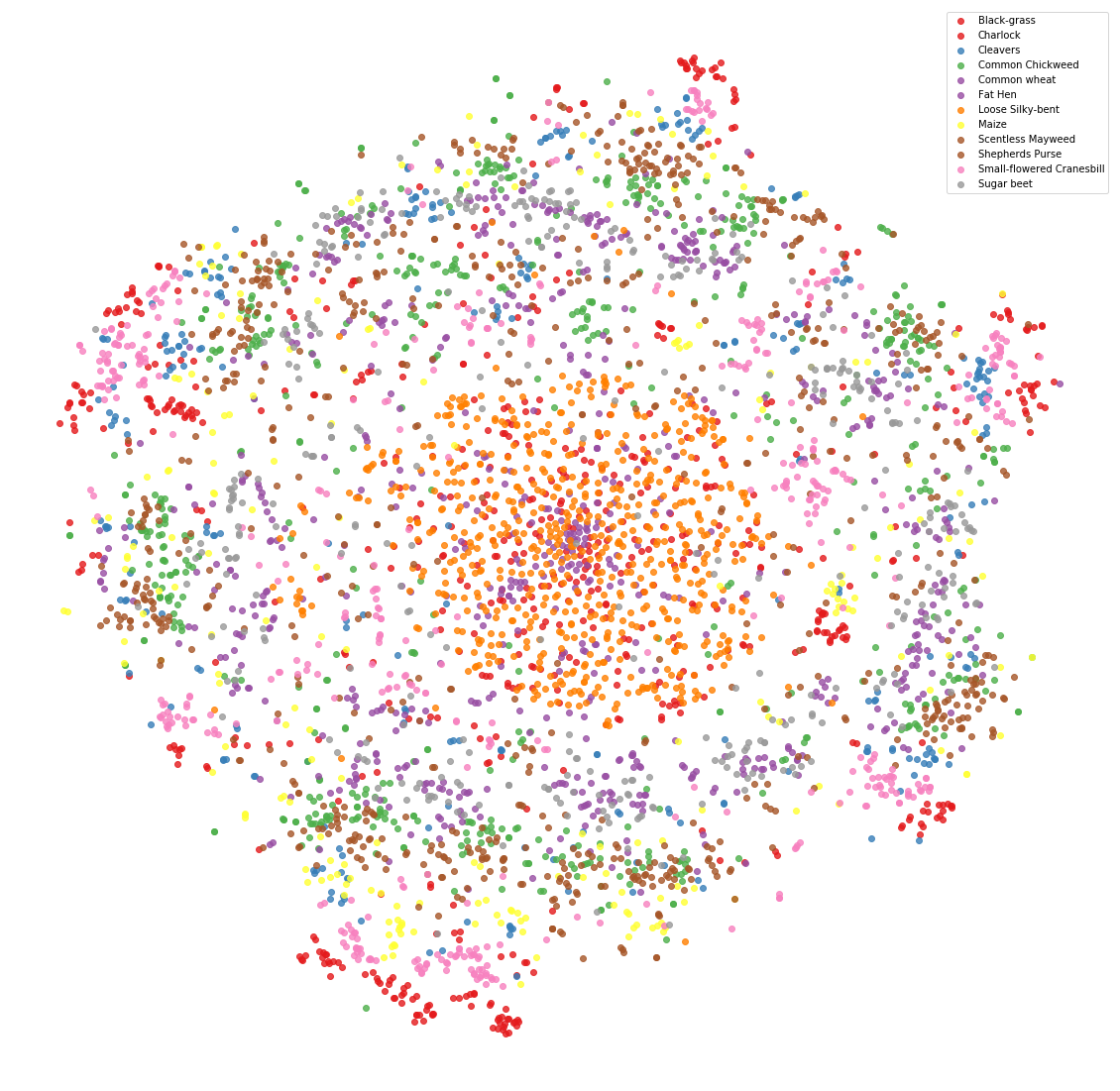展开全文• Python-matplotlib-隐藏坐标轴时图片没有数据显示 在《Python编程-从入门到实践》-【美】Eric Matthes 著，一书中对于随机漫步5000点的项目，为了避免坐标轴干扰对随机漫步路径的注意，需要隐藏坐标轴。 根据代码...
Python-matplotlib-隐藏坐标轴时图片没有数据显示
在《Python编程-从入门到实践》-【美】Eric Matthes 著，一书中对于随机漫步5000点的项目，为了避免坐标轴干扰对随机漫步路径的注意，需要隐藏坐标轴。
根据代码操作隐藏坐标轴，结果原本有数据的图片变成空白了。
代码如下：
while True:
# 创建一个RandomWalk实例，并将其包含的点都绘制出来
rw = RandomWalk(1000)
rw.fill_walk()
point_numbers = list(range(rw.num_points))
plt.scatter(rw.x_values, rw.y_values, c=point_numbers, cmap=plt.cm.Blues, edgecolor='none', s=15)
# 突出起点和终点
plt.scatter(0, 0, c='green', edgecolors='none', s=100)
plt.scatter(rw.x_values[-1], rw.y_values[-1], c='red', edgecolors='none', s=100)
# 隐藏坐标轴
plt.axes().get_xaxis().set_visible(False)
plt.axes().get_yaxis().set_visible(False)
plt.show()
keep_running = input("Make another walk? (y/n): ")
if keep_running == 'n':
break

将下面这两句隐藏坐标轴的语句注释掉，是有数据显示的，如下图
    plt.axes().get_xaxis().set_visible(False)
plt.axes().get_yaxis().set_visible(False)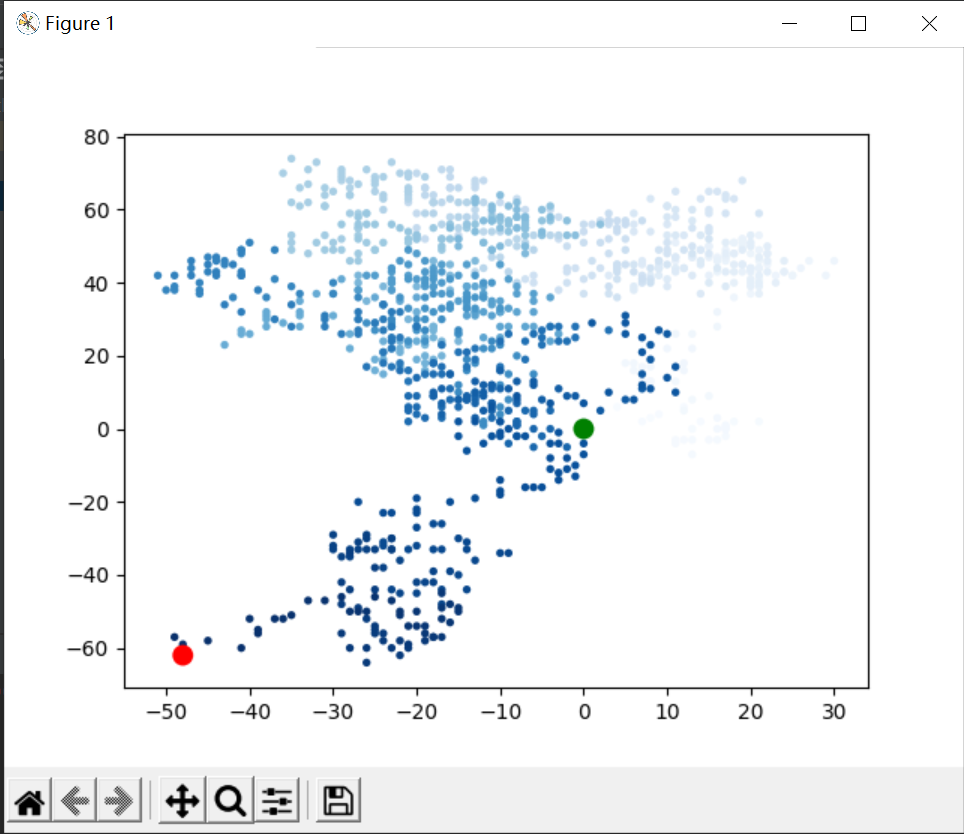将这两句取消注释后，图片变成空白了，没有数据显示，如下图。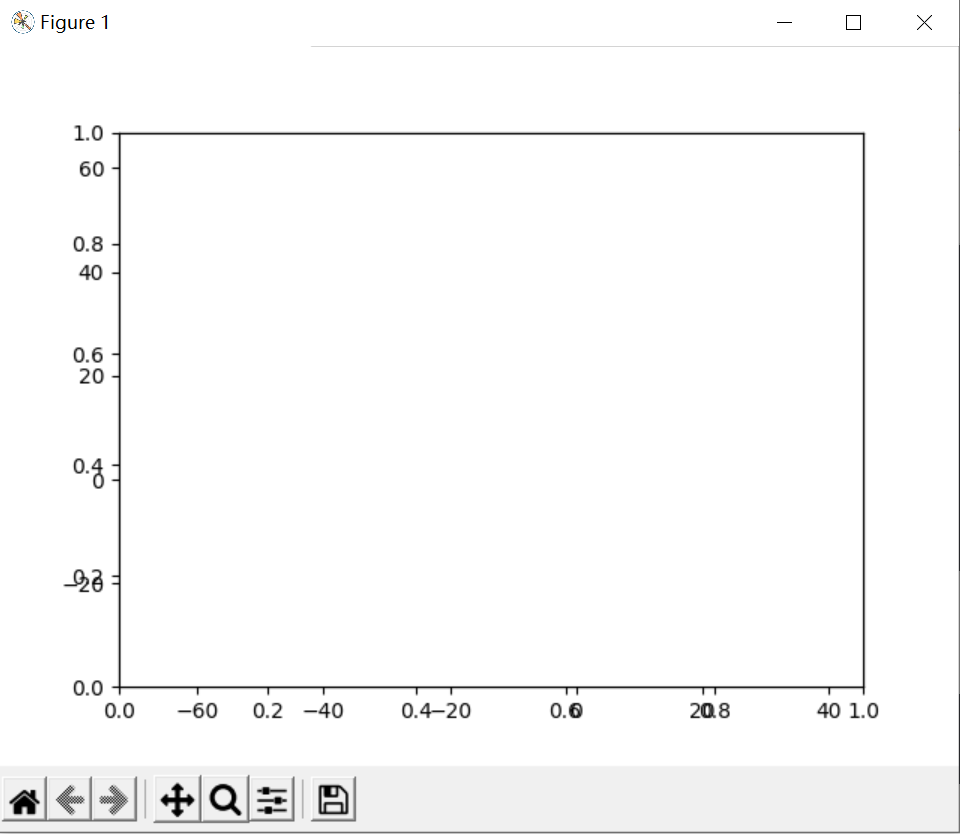代码修改如下：
while True:
# 创建一个RandomWalk实例，并将其包含的点都绘制出来
rw = RandomWalk(1000)
rw.fill_walk()

# # 隐藏坐标轴
# plt.axes().get_xaxis().set_visible(False)
# plt.axes().get_yaxis().set_visible(False)
current_axes = plt.axes()
current_axes.xaxis.set_visible(False)
current_axes.yaxis.set_visible(False)

point_numbers = list(range(rw.num_points))
plt.scatter(rw.x_values, rw.y_values, c=point_numbers, cmap=plt.cm.Blues, edgecolor='none', s=15)
# 突出起点和终点
plt.scatter(0, 0, c='green', edgecolors='none', s=100)
plt.scatter(rw.x_values[-1], rw.y_values[-1], c='red', edgecolors='none', s=100)
plt.show()

keep_running = input("Make another walk? (y/n): ")
if keep_running == 'n':
break
修改了两处地方：
1修改了隐藏坐标轴的代码：
    current_axes = plt.axes()
current_axes.xaxis.set_visible(False)
current_axes.yaxis.set_visible(False)
2修改了代码的位置，将隐藏坐标轴的代码放在了plt.show()的前面几行的位置。
只修改一个地方不能解决问题。
改后的效果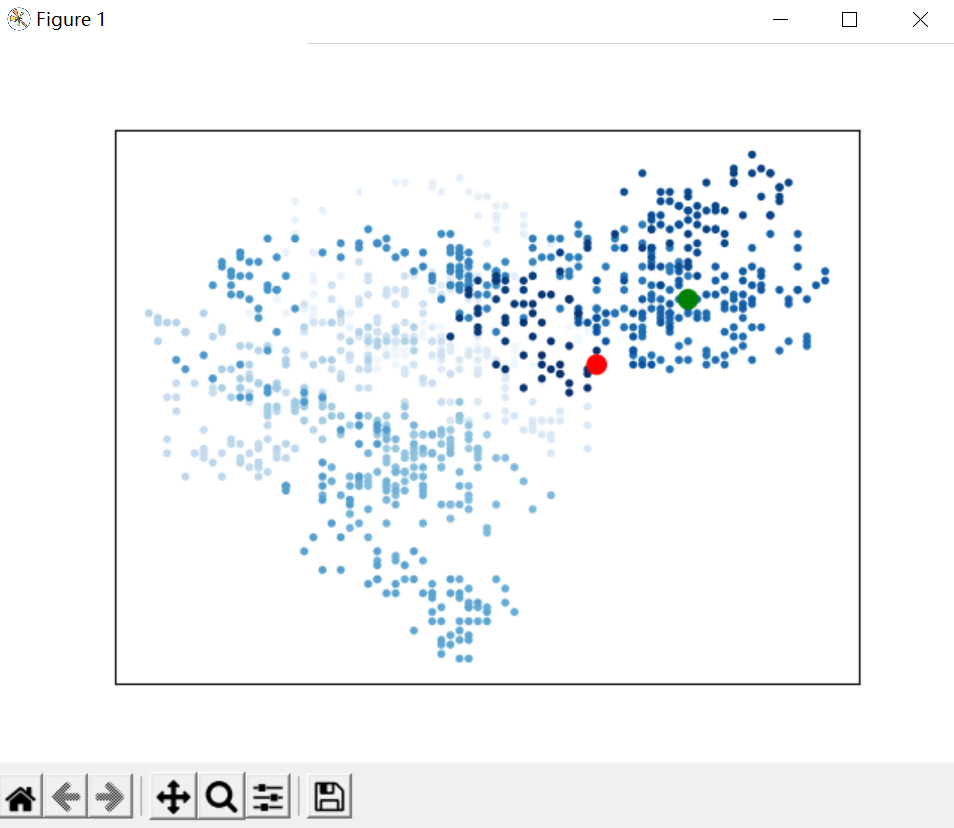展开全文python
• 文章目录一、默认情况下是显示坐标轴的二、怎样才能不显示坐标轴？ 一、默认情况下是显示坐标轴的 实例代码： import matplotlib.pyplot as plt from pylab import * Ux1 = [0.0227,1.8713,3.8285,6.5266,8.2801,9....python 开发语言 后端
• plt.xticks([]) # 去x坐标刻度 plt.yticks([]) # 去y坐标刻度 plt.axis('off') # 去坐标轴 **注意：**这三行代码要放到plt.show() 之前，plt.imshow() 之后
• 本文实例讲述了Python使用Matplotlib模块时坐标轴标题中文及各种特殊符号显示方法。分享给大家供大家参考，具体如下： Matplotlib中文显示问题——用例子说明问题 #-*- coding: utf-8 -*- from pylab import * t = ...
• importmatplotlib.pyplotasplt fromrandom_walkimportRandomWalk #只要程序处于活动状态，就不断地模拟随机漫步 whileTrue: #创建一个RandomWalk实例，并将其包含的点都绘制出来 rw=RandomWalk() rw....
• #! usr/bin/python #coding=utf-8 ...import matplotlib.pyplot as plt data=np.random.rand(10,10) fig, ax=plt.subplots() data[data==-1]=np.nan#去掉缺省值-1 im =ax.imshow(data,interpolation='npython
• 在《Python编程-从入门到实践》-【美】Eric Matthes 著，一书中对于随机漫步5000点的项目，为了避免坐标轴干扰对随机漫步路径的注意，需要隐藏坐标轴。 出现问题： 根据代码操作隐藏坐标轴，结果坐标不仅健在，还...python
• 容易的事。例如下面的代码： 绘制结果为： ...虽然确实几行代码就画出了正弦曲线，但是...虽然这个axisartist能够很方便地自定义坐标轴的位置和完美地设置箭头，但对于更多属性的设置有点乏力，很多参数的微调还...python 可视化
• plt.gray()：只有黑白两色，没有中间的渐进色 ... 关闭坐标轴： plt.axis('off') 注意，类似的这些操作若想起作用，需要将其置于 plt.show() 之前，plt.imshow() 之后。   对于 ax（matplot...
• 下面来介绍第二种方法实现三Y显示，这种方法是采用隐藏刻度的方式来实现的，先来看一下图： 可以看到这个图里有四个边线，叫做spine，也就是X、第一个Y、第二个Y和最上面的X。如果我们想要第三个Y，...python
• 使用Matplotlib绘制的图表的默认坐标轴是在左下角的，这样对于一些函数的显示不是非常方便，要改变坐标轴的默认显示方式主要要使用gca()方法 plt.gca()表示 Get current axis，使用这个方法我们可以获得整张图表的...
• 设置axes脊柱(坐标系) 属性列表 (1)去掉脊柱(坐标系) ax.spines[‘top’].set_visible(False) #去掉上边框 ax.spines[‘bottom’].set_visible(False) #去掉下边框 ax.spines[‘left’].set_visible(False) #去掉...
• 本想利用plt.xticks(tick[::60],list(tick.keys())[::60]),修改坐标轴的方法，但发现y轴数据有重合，效果不理想。 经查找资料发现一个好方法，但是该方法代码，我用起来有一定问题，可能是因为版本问题。 #原代码 ...python
• from random import choice import matplotlib.pyplot as plt x_start =  y_start =  #生成坐标矩阵 while len(x_start)<10000: x_direction=choice([-1,1]) x_distance=choice([0,1,2,3,4]) x_s...
• 的颜色及隐藏轴边框 import pandas as pd import matplotlib.pyplot as plt # 遇到数据中有中文的时候，一定要先设置中文字体 plt.rcParams['font.sans-serif'] = ['SimHei'] # 用黑体显示中文 x,y = plt.subplots...数据可视化
• 这就造成了一个问题，合并后的子图的部分坐标轴被掩盖，部分坐标轴裸露在外。如下图： 解决方案： 尝试一：通过ax.set_xticks([])方法设置坐标刻度不可见 ===> 效果：所有子图的坐标刻度都不可见，不满足设置单...
• 学习https://matplotlib.org/gallery/index.html ...import matplotlib.pyplot as plt plt.rcParams['font.sans-serif']=['SimHei'] # 用来正常显示中文标签 plt.rcParams['axes.unicode_minus']=False # 用来正常显...python绘图
• 隐藏所有刻度线和标签 plt.axis('off') 隐藏刻度线 默认左下True 右上False plt.tick_params(bottom=False, top=False, left=False, right=False) 隐藏边线 ax = plt.gca() for i in ['top', 'right', 'bottom',...
• matplotlib 可视化 —— 移动坐标轴（中心位置）
• 前面学习过隐藏坐标轴的方式来实现三Y轴的显示，并没有移动过坐标轴的位置，但是有时候需要移动坐标到某些位置，这样看曲线更加方便，比如像下图： 这时看到抛物线曲线分布在Y轴的两边，Y轴就是一条对称轴，分析...python
• 问题 我们在用matplotlib绘制图的时候总是有各种需求，...这里要介绍matplotlib绘图辅助工具包mpl_toolkits，里面包含了一系列对于坐标轴设置的方法。 首先导入辅助工具包里面的轴系设置包 import numpy as np imp...Python 程序员 编程
• 1. 去坐标轴： plt.axis(‘off‘) 2.去刻度： plt.xticks([]) plt.yticks([]) 3.执行上述操作时，需要将其置于 plt.show() 之前，plt.imshow() 之后。...